Click to Chat

1800-1023-196

+91-120-4616500

CART 0

• 0

MY CART (5)

Use Coupon: CART20 and get 20% off on all online Study Material

ITEM
DETAILS
MRP
DISCOUNT
FINAL PRICE
Total Price: Rs.

There are no items in this cart.
Continue ShoppingAcids and Bases

Table of Content

Arrhenius Concept

Limitations of Arrhenius concept

Bronsted-Lowry Concept - The Proton-Donor-Acceptor Concept

Lewis Concept of Acids and Bases

Lewis Acid &  Lewis Base

Limitations of Lewis Concept of Acid and Base

pH of Acids and Bases

pH of Weak Acids and Bases

Limitations of pH Scale

pH of Mixture

Periodic Variations of Acidic and Basic Properties

Hydracids of the Elements of the Same Periods

Hydracids of the Elements of Same Group

Oxyacids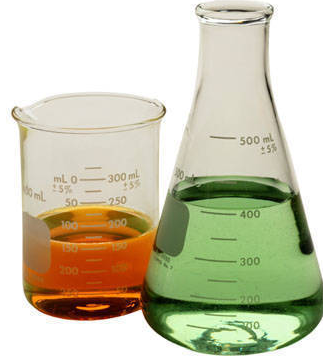The earliest criteria for the characterization of acids and bases were the experimentally observed properties of aqueous solutions. An acid  was defined as a substance whose water solution tastes sour, turns blue litmus red, neutralizes bases and so on. A substance was a base if its aqueous solution tasted bitter, turns red litmus blue, neutralizes acids and so on.

Faraday termed acids, bases and salts as electrolytes and Liebig proposed that acids are compounds containing hydrogen that can be replaced by metals. Different concepts have been put forth by different investigators to characterize acids and bases but the following are the three important modern concepts of acids and bases:

Arrhenius Concept

According to Arrhenius concept all substances which give H+ ions when dissolved in water are called acids while those which ionize in water to furnish OH- ions are called bases.

HA   H+ + A- (Acid)

BOH  B+ + OH- (Base)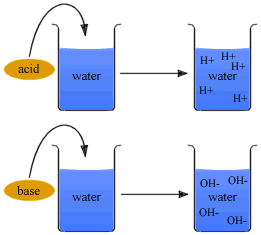Thus, HCl is an acid because it gives H+ ions in water. similarly, NaOH is a base as it yields OH- ions in water.

HCl  H+ + Cl-

NaOH   Na+ + OH-

Some acids and bases ionize completely in solutions and are called string acids and bases. Others are dissociated to a limited extent in solutions and are termed weak acids and bases. HCl, HNO3, H2SO4, HCIO4, etc., are examples of strong acids and NaOH, KOH, (CH3)4NOH are strong bases.

Every hydrogen compound cannot be regarded as an acid, e.g., CH4 is not an acid. Similarly, CH3OH, C2H5OH, etc., have OH groups but they are not bases, they must generate H+ or OH- ions in aqueous solution in order to be defined as acid or base

Actually free H+ ions are highly reaction so they do not exist in water. They combine with water molecules to form hydronium ion (H3O+) molecules, i.e., have strong tendency to get hydrated.

HX + H2O  H3O+ + X-
(Hydronium ion)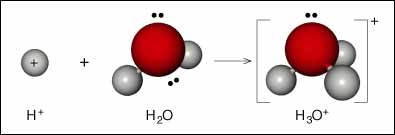The proton in aqueous solution is generally represented as H+ (aq). It is now known that almost all the ion are hydrated to more or less extent and it is customary to put (aq) after each ion.

The oxides of many non-metals react with water to form acids and are called acidic oxides or acid anhydrides.

CO2 + H2O  H2CO3  2H+(aq) +  (aq)

N2O5 + H2O  2HNO3  2H+(aq) +  (aq)

Many oxides of metals dissolve in water to form hydroxides. Such oxides are termed basic oxides.

Na2O + H2O →  2NaOH    2Na+(aq) + 2OH- (aq)

The substance like NH3 and N2H4 act as bases as they react with water to produce OH- ions.

NH3 + H2O →  NH4OH    NH+4 (aq) + OH- (aq)

The reaction between an acid and a base is termed neutralization. According to Arrhenius concept, the neutralization in aqueous solution involves the reaction between H+ and OH- ions or hydronium and OH-. This can be represented as

H3O+ + OH-   2H2O

Limitations of Arrhenius Concept

For the acidic or basic properties, the presence of water is absolutely necessary. Dry HCl shall not act as an acid. HCl is regarded as an acid only when dissolved in water and not in any other solvent.

The concept does not explain acidic and basic character of substances in non-aqueous solvents.

The neutralization process is limited to those reactions which can occur in aqueous solutions only, although reactions involving salt formation do occur in the absence of solvent.

It cannot explain the acidic character of certain salts such as AlCl3 in aqueous solution.

An artificial explanation is required to explain the basic nature of NH3 and metallic oxides and acidic nature of non-metal oxides.

Bronsted-Lowry Concept - The Proton-Donor-Acceptor Concept

Refer to the following video for Bronsted-Lowry concept

In 1923, Bronsted and Lowry independently proposed a broader concept of acids and bases. According to Bronsted-Lowry concept an acid is a substance (molecule or ion) that can donate proton, i.e., a hydrogen ion, H+, to some other substance and a base is a substance that can accept a proton from an acid. More simply, an acid is a proton donor (protogenic) and a base is a proton acceptor (protophilic).

Consider the reaction,

HCl + H2O   H3O + Cl-

In this reaction HCl acts as an acid because it donates a proton to the water molecule. Water, on the other hand, behaves as a base by accepting a proton from the acid.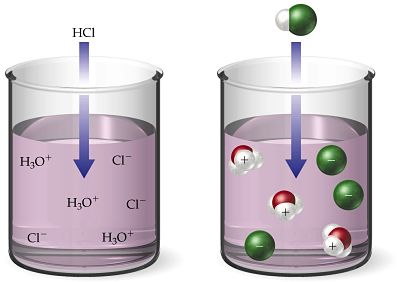The dissolution of ammonia in water may be represented as

NH3 + H2O  NH4+ + OH-

In this, reaction, H2O acts as an acid it donated a proton to NH3 molecule and NH3molecule behaves as a base as it accepts a proton.

When an acid loses a proton, the residual part of it has a tendency to regain a proton. Therefore, it behaves as a base.

Acid  H+ + Base

The acid and base which differ by a proton are known to form a conjugate pair. Consider the following reaction.

CH3COOH + H2O   H3O+ + CH3COO-

It involves two conjugate pairs. The acid-base pairs are: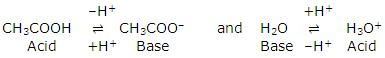Such pairs of substances which can be formed form one another by loss or gain of a proton are known as conjugate acid-base pairs.

If in the above reaction, the acid CH3COOH is labelled acid1 and its conjugate base, CH3COO- as base1. H2O is labelled as base2 and its conjugate acid H3O+ as acid2, the reaction can be written as:

Acid1 + Base2  Base1 + Acid2

Thus, any acid-base reaction involves two conjugate pairs, i.e., when an acid reacts with a base, another acid and base are formed.

Thus, every acid has its conjugate base and every base has its conjugate acid. It is further observed that strong acids have weak conjugate bases while weak acids have strong conjugate bases.

HCl        +           Cl-                CH3COOH     +   CH3COO-

Strong acid       Weak base      Weak acid        Strong base

There are certain molecules which have dual character of an acid and a base. These are called amphiprotic or atmospheric.

Examples are NH3, H2O, CH3COOH, etc.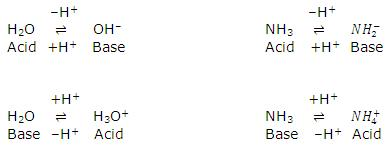The strength of an acid depends upon its tendency to lose its proton and the strength of the base depends upon its tendency to gain the proton.Acid-Base chart containing some common conjugate acid-base pairs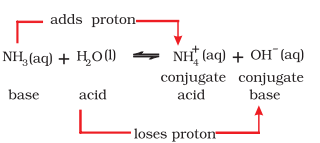Acid

Conjugate base

KClO4

(Perchloric acid)

(Perchlorate ion)

H2SO4

(Sulphuric acid)

(Hydrogen sulphate ion)

HCl

(Hydrogen chloride)

Cl-

(Chloride ion)

HNO3

(Nitric acid)

(Nitrate ion)

H3O+

(Hydronium ion)

H2O

(Water)

(Hydrogen sulphate ion)

(Sulphide ion)

H3PO4

(Ortho phosphoric acid)

H2

(Dihydrogen phosphate ion)

CH3COOH

(Acetic acid)

Ch3COO-

(Acetate ion)

H2CO3

(Carbonic acid)

(Hydrogen carbonate ion)

H2S

(Hydrogen sulphide)

(Hydorsulphide ion)

NH3+

(Ammonium ion)

NH3

(Ammonia)

HCN

(Hydrogen cyanide)

CN-

(Cyanide ion)

C6H5OH

(Ethyl alcohol)

C6H5O-

(Phenoxide ion)

NH3

(Ammonia)

OH-

(Hydroxide ion)

CH4

(Methane)

C2H5O-

(Ethoxide ion)

In acid-base strength series, all acids above H3O+ in aqueous solution fall to the strength of H3O+. Similarly the basic strength of bases below OH- fall to the strength of OH- in aqueous solution. This is known as leveling effect.

The strength of an acid also depends upon the solvent. The acids HCIO4, H2S04, HCl and HN03 which have nearly the same strength in water will be in the order of HClO4> H2SO4 > HCl > HNO3 in acetic acid, since the proton accepting ten­dency of acetic acid is much weaker than water. So the real strength of acids can be judged by solvents. On the basis of proton interaction, solvents can be classified into four types:

Protophilic solvents:  Solvents which have greater tendency to accept protons, i.e., water, alcohol, liquid ammonia, etc.

Protogenic solvents:  Solvents which have the tendency to produce protons, i.e., water, liquid hydrogen chloride, glacial acetic acid, etc.

Amphiprotic solvents:  Solvents which act both as protophilic or protogenic, e.g., water, ammonia, ethyl alcohol, etc.

Aprotic solvents:  Solvents which neither donate nor accept protons, e.g., benzene, carbon tetrachloride, carbon disulphide, etc.

HCI acts as acid in H3O+, stronger acid in NH3, weak acid in CH3COOH, neutral in C6H6and a weak base in HF.

HCI   +   HF     →    H2Cl+  +   F-

Base      Acid          Acid             Base

Lewis Concept of Acids and Bases

?Refer to the following video for Lewis concept of acids and bases

This concept was proposed by G.N. Lewis, in 1939. Accord­ing to lewis definition of acids and bases base is defined as a substance which can furnish a pair of electrons to form a coordinate bond whereas an acid is a substance which can accept a pair of electrons. The acid is also known as electron acceptor or electrophile while the base is electron donor or nucleophile.

A simple example of an acid-base is the reaction of a proton with hydroxyl ion.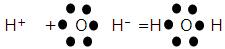Acid        Base

Some other examples are:

H3N: + BF3 = H3N → BF3

Base   Acid

H+ +: NH3 = [H ← NH3]+

Acid    Base

BF3 + [F]- = [F → BF3]+

Acid  Base

Lewis concept is more general than the Bronsted Lowry concept.

According to Lewis concept, the following species can act as Lewis acids.

Molecules in which the central atom has incomplete octet: All compounds having central atom with less than 8 electrons are Lewis acids, e.g., BF3, BC13, A1C13, MgCl2. BeCL. etc.

Simple cations: All cations are expected to act as Lewis acids since they are deficient in electrons. However, cations such as Na+, K+, Ca2+, etc., have a very little tendency to accept electrons, while the cations like H+, Ag+, etc., have greater tendency to accept electrons and, therefore, act asLewis acids.

Molecules in which the central atom has empty d-orbitals: The central atom of the halides such as SiX4, GeX4, TiCl4, SnX4, PX3, PF5, SF4, SeF4, TeCl4, etc., have vacant d-orbitals. These can, therefore, accept an electron pair and act as Lewis acids.

Molecules having a multiple bond between atoms of dissimilar electronegativity: Typical examples of molecules falling in this class of Lewis acids are C02, S02 and S03. Under the influence of attacking Lewis base, one -electron pair will be shifted towards the more negative atom.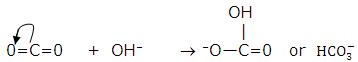Lewis Acid &  Lewis Base

The following species can act as Lewis bases.

Neutral species having at least one lone pair of electrons: For example, ammonia, amines, alcohols, etc., act as Lewis bases because they contain a pair of electrons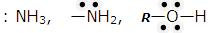Negatively charged species or anions: For example, chloride, cyanide, hydroxide ions, etc., act as Lewis bases.CN-,   CI-,  OH-

It may be noted that all Bronsted bases are also Lewis bases but all Bronsted acids are not Lewis acids.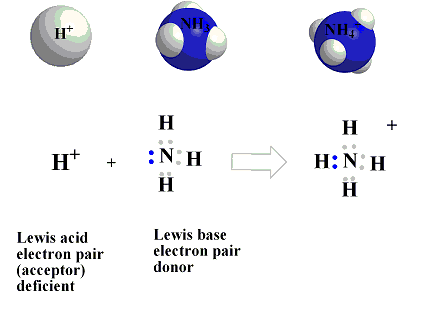Limitations of Lewis Concept of Acid and Base

Since the strength of the Lewis acids and bases is found to depend on the type of reaction, it is not possible to arrange them in any order of their relative strength.

The choice of which definition of acids and bases one wishes to use in a particular instance depends largely on the sort of chemistry that is studied. But Arrhenius concept is perfectly satisfactory and simplest for dealing with reactions in aqueous solutions. It explains satisfactorily the strength of acids and bases in aqueous solutions, neutralisation, salt hydrolysis, etc.

pH of Acids and Bases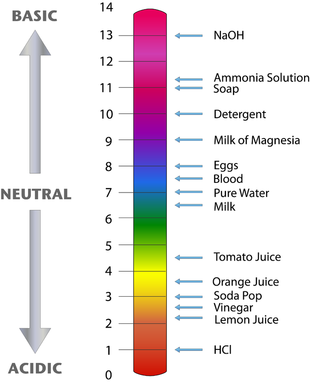It is clear from the above discussion that nature of the solution (acidic, alkaline or neutral) can be represented in terms of either hydrogen ion concentration or hydroxyl ion concentration but it is convenient to express acidity or alkalinity of a solution by referring to the concentration of hydrogen ions only. pH measurement is done using pH paper and pH meter

Since H+ ion concentration can vary within a wide range from 1 mol per litre to about 1.0 × 10-14mol per litre, a logarithmic notation has been devised by Sorensen, in 1909, to simplify the expression of these quantities. The notation used is termed as the pH scale.

The hydrogen ion concentrations are expressed in terms of the numerical value of negative power to which 10 must be raised. This numerical value of negative power was termed as pH, i.e.,

pH  = – log aH+ (Where aH+ is the activity of H+ ions).

Activity of H+ ions is the concentration of free H+ ions in a solution. By free, we mean those that are at a large distance from the other ion so as not to experience  its pull. We can infer from this that in dilute solutions, the activity of an ion is same as its concentration since more number of solvent molecules would separate the two ions. For concentrated solutions the activity would be much less than the concentration itself.

Therefore, the earlier given expression of pH can be modified for dilute solutions as,
pH  = – log [H+].

This assumption can only be made when the solution is very much dilute, i.e, [H+]  1M. For higher concentration of H+ ions, one needs to calculate the activity experimentally and then calculate the pH.

pH of a solution is, thus, defined as the negative logarithm of the concentration (in mol per litre) of hydrogen ions which it contains or pH of the solution is the logarithm of the reciprocal of H+ ion concentration.

Just as pH indicates the hydrogen ion concentration, the pOH represents the hydroxyl ion concentration, i.e.,

pOH = -log [OH-] Considering the relationship,

[H+][OH-] = Kw = 1 x 10-14

Taking log on both sides, we have

log [H+] + log [OH-] = log Kw = log (1 x 10-14)

or -log [H+] - log [OH-] = -log Kw = -log (1 x 10-14)

or    pH + pOH = PKw* = 14

i.e., sum of pH and pOH is equal to 14 in any aqueous solution at 25°C. The above discussion can be summarised in the following manner:

[H+]

[OH-]

pH

pOH

Acidic solution

Neutral solution

Basic solution

>10-7

10-7

<10-7

<10-7

10-7

>10-7

<7

7

>7

>7

7

<7

[H+]

[OH-]

pH

pOH

Nature of solution

100

10-2

10-5

10-7

10-9

10-11

10-14

10-14

10-12

10-9

10-7

10-5

10-3

10-0

0

2

5

7

9

11

14

14

12

9

7

5

3

0

Strongly acidic Acidic

Weakly acidic

Neutral

Weakly basic

Basic

Strongly basic

pH of Weak Acids and Bases

Weak acids and bases are not completely ionised; an equilibrium is found to have been established between ions and unionised molecule. Let us consider a weak acid of basicity 'n'.

AHn    An-  +   nH+

-C        0          0

teq      C(1-α)   Cα       nCα

[H+] = nCα;  .·. pH = -log10 [nCα]

For monobasic and,  n=1

pH = -log10 [Cα]

Dissociation constant of acid Ka may be calculated as

Ka = [An-][H+]n/[AHn] = [Cα][nCα]n/[C(1-α)]

=  α [nCα]n/(1-α)           For weak acids, α« 1

.·. (1-α) = 1

= α[nCα ]n/(1-α)

nCKa = nCα [nCα ]n = [nCα ](n+1)

= [nCα ] = [nCKa]1/(n+1)

= [H+] = [nCKa]1/(n+1)

.·. pH = -1/(n+1) log10(nCKa)

For monobasic acid, n = 1

pH = -log√CKα

Since Ka = α[nCα]n

ka/α = (nCα)n

[nCα ] = [Kα/α]1/n = [H+]

pH = -1/n log10(Kα/α)

For n = 1       pH = -log10(Kα/α)

Limitations of pH Scale

pH values of the solutions do not give us immediate idea of the relative strengths of the solutions. A solution of pH = 1 has a hydrogen ion concentra­tion 100 times that of a solution of pH = 3 (not three times). A 4 x 10-5 N HCI is twice concentrated of a 2 x 10-5 N HCI solution, but the pH values of these solutions are 4.40 and 4.70 (not double).

pH value of zero is obtained in 1 A' solution of strong acid. In case the concentration is 2 N, 3 N, 10 N, etc. The respective pH values will be negative.

A solution of an acid having very low concentration, say 10-8 N, cannot have pH 8, as shown by pH formula, but the actual pH value will be less than 7.

Note:

Normality of strong acid = [H3O+]
Normality of strong base = [OH-]
.·. pH = -log [N]    for strong acids
pOH = -log [N]    for strong acids

Sometimes pH of acid comes more than 7 and that of base comes less than 7. It shows that the solution is very dilute; in such cases, H+ or OH- contribution from water is also considered, e.g., in 10   N HCI,

[H+]Total = [10-8]Acid + [10-7]Water

= 11 × 10-8 M= 1.1 × 10-7 M

pH of Mixture

Let one litre of an acidic solution of pH 2 be mixed with two litre of other acidic solution of pH 3. The resultant pH of the mixture can be evaluated in the following way.

Sample 1

Sample 2

pH = 2

pH = 3

[H+]=10-2 M

[H+] = 10-3M

V = 1 litre

V= 2 litre

MlVl+M2V2 = MR(Vi + V2) 10-2 × 1 + 10-3 × 2 = MR (1 +2)

(12 + 10-3)/3  = MR

4 × 10-3 = MR(Here, MR = Resultant molarity)

pH = -log (4 × 10-3)

Total concentration of [H+] or  [H3+O ] in a mixture of weak acid and a strong acid  = (C2 + √(c22 + 4KaC1 ))/2

where C1 is the concentration of weak acid (in mol litre having dissociation constantKa

C2 is the concentration of strong acid

Total [OH-] concentration in a mixture of two weak bases =   √(K1C1+ K2C2)

where K1 and K2 are dissociation constants of two weak bases having C1 and C2 as their mol litre-1 concentration respectively.

Periodic Variations of Acidic and Basic Properties

(a)  Hydracids of the Elements of the Same Periods

Consider the hydracids of the elements of II period, Viz., CH4, NH3, H20 and HF. These hydrides become increasingly acidic as we move from CH4 to HF. CH4 has negligible acidic properties while HF is a fairly stronger acid. The increase in acidic properties is due to the fact that the stability of their conjugate bases increases in the order

CH-3< NH-2  < OH- < F-

The increase in acidic properties is supported by the succes­sive increase in the dissociation constant.

CH4(=10-58)<NH3 (=10-35)<H20(=10-14)<HF(=10-4)

(b)  Hydracids of the Elements of Same Group

Hydrides of V group elements (NH3, PH3, AsH3, SbH3, BiH3) show basic character which decreases due to increase in size and decrease in electronegativity from N to Bi. There is a decrease in electron density in, sp3 -hybrid orbital and thus electron donor capacity decreases.

Hydracids of VI group elements (H20, H2S, H2Se, H2Te) act as weak acids. The strength increases in the order H20 < H2S < H2Se < H2Te. The increasing acidic properties reflects decreasing trend in the electron donor capacity of OH-, HS-, HSe- or HTe- ions.

Hydracids of VII group elements (HF, HCI, HBr, HI) show acidic properties which increase from HF to HI. This is explained by the fact that bond energies decrease. (H-F = 135 kcal/mol, HCI = 103, HBr = 88 and HI = 71 kcal/mol).

(c) Oxyacids

The acidic properties of oxyacids of the same element which is in different oxidation states increases with increase in oxidation number.

+ 1            +3          +5            +7

HCIO  <  HC1O2   <   HC1O3   <  HCIO4

+4            +6         +3            +5

H2SO3  <   H2SO4;    HNO2     <  HNO3

But this rule fails in oxyacids of phosphorus.

H3PO2  >  H3PO3  >  H3PO4

The acidic properties of the oxyacids of different elements which are in the same oxidation state decreases as the atomic number increases. This is due to increase in size and decrease in electronegativity.

HC1O4 > HBrO4 > HIO4

H2SO3  > H2SeO3

But there are a number of acid-base reactions in which no proton transfer takes place, e.g.,

SO2 + SO2  ↔  SO2+ +  S

Acid1  Base2    Acid2   Base1

Thus, the protonic definition cannot be used to explain the reactions occurring in non-protonic solvents such as COCl2, S02, N2O4, etc.Example 1:  The hydrogen ion concentration of a solution is 0.001 M. What will be the hydroxyl ion concentration of solution?

Solution: We know that [H+][OH-] 1.0 × 10-14

Given that,

[H+] = 0.001 M = 10-3 M

So, [OH-] = 1.0 * 10-14/[H+] = (1* 10-14)/10-3 = 10-11M

Example 2:  What is the pH of the following solutions?

(a) 10-3 M HCl

(b) 0.0001 M NaOH

(c)  0.0001 MH2S04

Solution:   HCI is a strong electrolyte and is completely ionised.

HCl  H+ + CI-

So   [H+] = 10-3 M

pH = -log [H+] = -log (10-3) = 3

(b)   NaOH is a strong electrolyte and is completely ionised.

NaOH ↔ Na+ + OH-
So    [OH+] = 0.0001 M = 10-4 M

pOH = -log(10-4) = 4

As   pH + pOH = 14

So   pH + 4 = 14   or     pH=10

Alternative method:   [OH-] = 10-4 M

We know that    [H+][OH-] = 1.0 x 10-14

So  [H+] = (1.0  10-14)/10-14

pH = -log [H+] = -log(10-10) = 10

(c) H2SO4 is a strong electrolyte and is ionized completely.

H2SO4 ↔ 2H+ + SO2-4

One molecule of H2SO4 furnishes ions.

So [H+] = 2 × 10-4 M

pH = -log [H+]

= -log (2×10-4)

= 3.70

Example 3: Find the pH of a 0.002 N acetic acid solution, if it is 2.3% ionised at a given dilution.

Solution: Degree of dissociation, α = 2.3/100 = 0.023

Concentration of acetic acid, C = 0.002 M

The equilibrium is

CH3COOH  CH3COO- + H+

C(1-α)         Cα           Cα

So  [H+] = Cα  = 0.002 × 0.023 = 4.6 × 10-5 M

pH = -log [H+]= -log (4.6 × 10-5) = 4.3372

Example 4:   Calculate the pH value of a solution obtained by mixing 50 mh of 0.2 N HCl with 50 mL of 0.1 N NaOH.

Solution:    Number of milli-equivalents of the acid

= 50 × 0.2 = 10

Number of milli-equivalents of the base

= 50 × 0.1 =5

Number of milli-equivalents of the acid left after the addition of base

= (10-5) = 5 Total volume of the solution

= 50 + 50 = 100 mL

Thus, 5 milli-equivalents of the acid are present in 100 mL of solution.

or 50 milli-equivalents of the acid are present in one litre of solution.

or 0.05 equivalents of the acid are present in one litre of solution.

The acid is monobasic and completely ionised in solution.

0.05 N HCl = 0.05 M HCl

So     [H+] = 0.05 M

pH = -log [H+] = -log 5 × 10-2 = -[log 5.0 + log 10-2]

= -[0.70-2] = 1.3

Example 5:  What will be the pH of a solution obtained by mixing 800 mL of 0.05 A' sodium hydroxide and 200 mL of OA N HCl, assuming complete ionisation of the acid and the base ?

Solution:   Number of milli-equivalents of NaOH

= 800 × 0.05 = 40

Number of milli-equivalents of HCl

= 200 × 0.1 =20

Number of milli-equivalents of NaOH left after the addition of HCl

= (40 -20) = 20

Total volume = (200 + 800) mL = 1000 mL = 1 litre

20 milli-equivalents or 0.02 equivalents of NaOH are present in one litre, i.e.,

0.02 N NaOH = 0.02 M NaOH (Mono-acidic) and the base is completely ionised.

So     [OH-] = 0.02 M

or     [OH-]= 2 × 10-2 M

pOH = -log(2 × 10-2) = 1.7

We know that,   pH + pOH = 14

So   pH = (14-1.7)= 12.3

Look here for

Chemistry Syllabus for IIT JEE

Best books of Chemistry.

To read more, Buy study materials of Chemical Equilibrium comprising study notes, revision notes, video lectures, previous year solved questions etc. Also browse for more study materials on Chemistry here.### Course Features

• 731 Video Lectures
• Revision Notes
• Previous Year Papers
• Mind Map
• Study Planner
• NCERT Solutions
• Discussion Forum
• Test paper with Video Solution